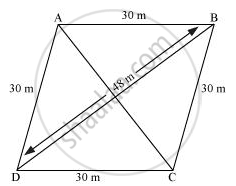# A rhombus shaped field has green grass for 18 cows to graze. If each side of the rhombus is 30 m and its longer diagonal is 48 m, how much area of grass field will each cow be getting? - Mathematics

A rhombus shaped field has green grass for 18 cows to graze. If each side of the rhombus is 30 m and its longer diagonal is 48 m, how much area of grass field will each cow be getting?

#### SolutionLet ABCD be a rhombus-shaped field.

For ΔBCD,

Semi-perimeter,

s=(48+30+30)/2 = 54 m

By Heron's Formula,

"Area of triangle "=sqrt(s(s-a)(s-b)(s-c))

therefore" Area of ΔBCD "=[sqrt(54(54-48)(54-30)(54-30))]m^2

=sqrt(54(6)(24)(24))

= 3 x 6 x 24

= 432 m2

Area of field = 2 × Area of ΔBCD

= (2 × 432) m2 = 864 m2

Area for grazing for 1 cow = 864/18 = 48 m2

Each cow will get 48 m2 area of grass field.

Concept: Application of Heron’s Formula in Finding Areas of Quadrilaterals
Is there an error in this question or solution?

#### APPEARS IN

NCERT Class 9 Maths
Chapter 12 Heron's Formula
Exercise 12.2 | Q 5 | Page 207